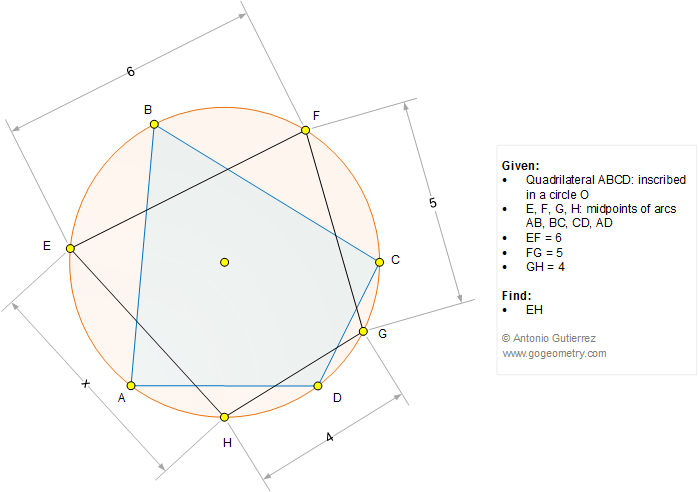# Geometry Problem 1245

### Elements: Cyclic or Inscribed Quadrilateral, Circle, Arc, Midpoint, Measurement. Mind Map

In the figure below, a quadrilateral ABCD is inscribed in a circle O. E, F< G, and H are the midpoints of arcs AB, BC, CD, and AD, respectively. If EF = 6, FG = 5, and GH = 4, find EH.## "How to Solve It" Mind Map

George Polya's 1945 book "How to Solve It, A new aspect of Mathematical Method", is a book describing methods of problem solving. It suggests the following steps when solving a mathematical problem: (1) First, you have to understand the problem. (2) After understanding, then make a plan. (3) Carry out the plan. (4) Look back on your work. How could it be better?